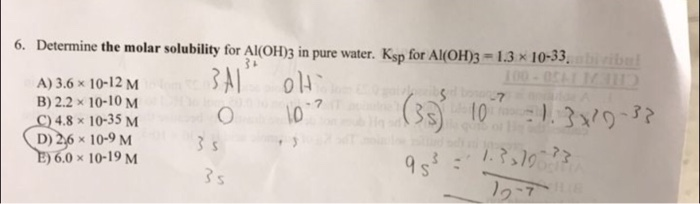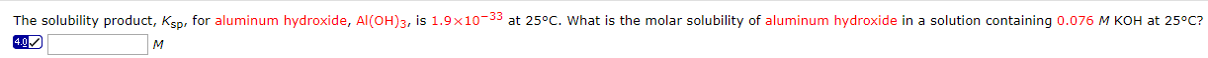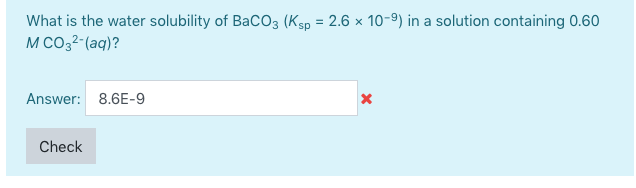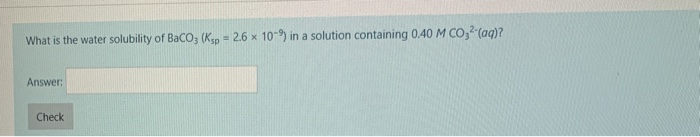# Determine the molar solubility for Al(OH)3 in pure water. Ksp for Al(OH)3 = 1.3 x 10-33 2.6 x 10-9 M is the answer,...

Determine the molar solubility for Al(OH)3 in pure water. Ksp for Al(OH)3 = 1.3 x 10-33

Use Al(OH)3(s) which has a Ksp = 1.3 x 10-33 M4

1. Write the chemical reaction.

Al(OH)3(s) <---> Al3+(aq) + 3OH-(aq)

2. Write the Ksp expression.

Ksp = [Al3+] [OH-]3

3. Let "s" equal the molar solubility of Al(OH)3(s).

From the reaction stoichiometry:

[Al3+] = s and [OH-] = 3s

Substitute into the Ksp expression:

```Ksp = (s)(3s)3 = 1.3 x 10-33 M4

(s)(27s3) = 1.3 x 10-33 M4

27s4  = 1.3 x 10-33 M4

s4 = 4.8 x 10-35 M4

s = 2.6 x 10-9 M
```
```
So   [Al3+] = 2.6 x 10-9 M```

and

[OH-] = 3(2.6 x 10-9 M) = 7.8 x 10-9 M

##### Add Answer of: Determine the molar solubility for Al(OH)3 in pure water. Ksp for Al(OH)3 = 1.3 x 10-33 2.6 x 10-9 M is the answer,...
Similar Homework Help Questions
• ### 07 6. Determine the molar solubility for Al(OH)3 in pure water. Ksp for Al(OH)3 = 1.3 x 10-33. A) 3.6 x 10-12 M Ol...07 6. Determine the molar solubility for Al(OH)3 in pure water. Ksp for Al(OH)3 = 1.3 x 10-33. A) 3.6 x 10-12 M Ol S 7 B) 2.2 x 10-10 M C) 4.8 x 10-35 M (D) 2,6 x 10-9 M E) 6,0 x 10-19 M 29-7 (3 109 21.370-3? 98151.7370-

• ### Determine the molar solubility of Al(OH)3 in a solution containing 0.0500M AlCl3, Ksp (Al(OH)3)=1.3*10^-33

Determine the molar solubility of Al(OH)3 in a solution containing 0.0500M AlCl3, Ksp (Al(OH)3)=1.3*10^-33

• ### Determine the molar solubility of Fe(OH)2 in pure water. Ksp for Fe(OH)2)= 4.87 Ã— 10-17. Please explain your answe...

Determine the molar solubility of Fe(OH)2 in pure water. Ksp for Fe(OH)2)= 4.87 Ã— 10-17. Please explain your answer and I will rate 5 stars! Thanks!

• ### Calculate the molar solubility of Mg(OH)2 in the following solvents. Ksp = 1.8 x 10¯11 pure...

Calculate the molar solubility of Mg(OH)2 in the following solvents. Ksp = 1.8 x 10¯11 pure water 8.68×10?2 M MgCl2 3.65×10?2 M KOH(aq). Really i just need someone to explain how these things affect solubility numerically

• ### Determine the molar solubility of PbSO4 in pure water. Ksp(PbSO4)= 1.82 x 10^-8.

Determine the molar solubility of PbSO4 in pure water. Ksp(PbSO4)= 1.82 x 10^-8.

• ### Determine the molar solubility of AgI in pure water. Ksp (AgI)= 8.51 x 10-17

Determine the molar solubility of AgI in pure water. Ksp (AgI)= 8.51 x 10-17

• ### Please give answer with correct sigfigs The solubility product, Ksp, for aluminum hydroxide, Al(OH)3, is 1.9x10-33...Please give answer with correct sigfigs The solubility product, Ksp, for aluminum hydroxide, Al(OH)3, is 1.9x10-33 at 25°C. What is the molar solubility of aluminum hydroxide in a solution containing 0.076 M KOH at 25°C? 4.0 M

• ### What is the water solubility of BaCO3 (Ksp = 2.6 x 10-9) in a solution containing...What is the water solubility of BaCO3 (Ksp = 2.6 x 10-9) in a solution containing 0.60 M CO32-(aq)? Answer: 8.6E-9 Check

• ### Determine the molar solubility of PbSO4 in pure water. Ksp (PbSO4) = 1.82 times 10-8.

Determine the molar solubility of PbSO4 in pure water. Ksp (PbSO4) = 1.82 times 10-8.

• ### What is the water solubility of Baco; (Ksp - 2.6 x 10-9 in a solution containing...What is the water solubility of Baco; (Ksp - 2.6 x 10-9 in a solution containing 040 M CO, (aq)? Answer: Check

Need Online Homework Help?